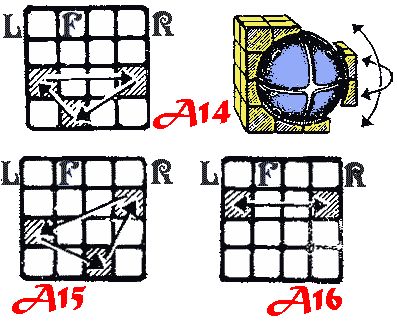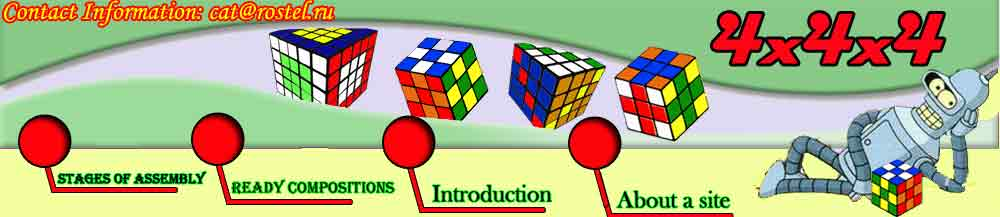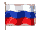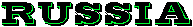Updating of a cube 3×3×3 is a cube 4×4×4, or "Master". In this puzzle the habitual cube has as though appeared cut on the center of sides on 4 parts. Instead of one there were two average layers, and operation of turn of an average layer "has forked". Instead of one central cube on each side became four. The device of the mechanism allowing cubes to move from a side on a side more complex. Instead of a cross the center of a cube-it is a sphere with grooves for moving the central cubes. Peripheral cubes which are kept central, slide directly on a surface of a sphere.For its description we use the designations accepted at us for a cube 3×3×3. Considering, that in a cube 4×4×4 instead of one there will be two average layers, operations of turn of an average layer here has changed, and Rc<>L'c (Cr<>C'l).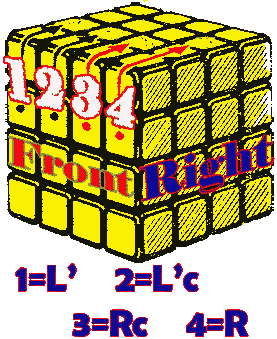1. The central cubes of a front side steal up process of moving of algorithm 1. The necessary cube should be preliminary deduced on the top side. (RRC) U' (R'R'C)................. А1
It is necessary to consider, that color of the central cubes should be defined on the chosen arrangement of angular and costal cubes, in pairs for sides F-B, U-D, R-L. It can appear, that color of the centers of last pair should be changed places. Algorithm А2 changes color of the center of sides F and B
[(UC)*2 (R*2) (L*2)]*2 - (U*2)(D*2).............. А2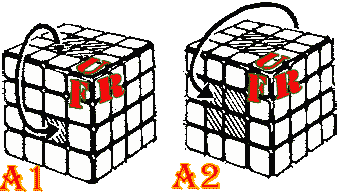2. Costal cubes. Four pairs costal cubes of the top side steal up by means of connection of two cubes in one-colour pair.Turn of average layers and moving of the restored pairs on a place by means of turn of external layers is made. In figure it is shown how to connect costal cubes fd or rd in pair fr. Algorithm А3 moves the necessary cubes from the bottom layer to average layers
R' D R and F D' F '........... А_31 and А_32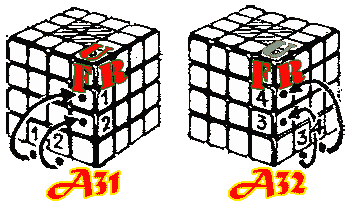Designations: R is there is a turn of the right side on 90 clockwise. (RC) is there is a turn of the right average layer. (RRC) is is turn of the right and right 2 average layers on 90 clockwise. (R*2) -it is turn on 180, double turn, L ' -it is turn of the left side on 90 counter-clockwise. (L'C) is there is a turn of the left average, etc. Letters F, U, B, D designate accordingly turns of front, up, back, down sides. C=Center

The third stage: the selection of 3 angular cubes of the top side does not differ from similar operations with a cube 3*3*3.
F D F '......... А_41
F ' D ' F....... А_42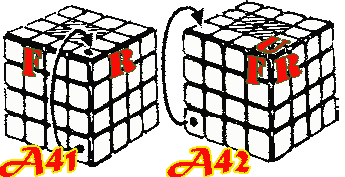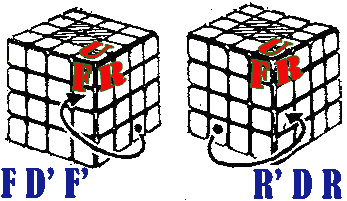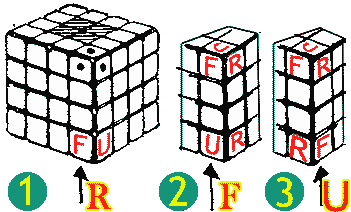4. The fourth stage. The selection of six costal cubes of a belt (two average layers) Process occurs by means of turns U, UC, D, DC and operations F D ' F ' and R ' D R. The free corner of the top side is used. First it is necessary to pick up on color three costal cubes on top average layer UC. It is necessary to find a costal cube on the bottom side and to translate it on top layer UC (look figure). If operations F D ' F ' and R ' D R do not lead to the purpose you have chosen not that cube which is necessary, and its "double" who should pass in the bottom layer. The fourth cube yet do not install, leaving a free corner. As select three costal cubes of the bottom layer. Establish free angular and costal cubes from layers U and UC and the necessary cube in one layer. Then execute operations of moving from below to the right or from below on the left, that is.......... F D ' F ' or R ' D R - А3 (algorithm А3).

The fifth stage. Installation of two remained costal cubes of average layers. Put a cube in layer UC and restore the center (the description is given above). Then turn of the bottom side to bring a cube. It will come in one of two conditions shown in figure. Algorithm А5 translates from position №2 in position №1. Algorithm А6 establishes last costal cube on a place.
D R D - F D' F R' .................................   А5
R (UC U)    L D' L' D (U'U'C)  R'........... А6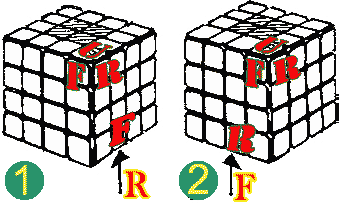6. The sixth stage. Installation of the fourth angular cube of the top side.
Process
№1 [ D F ' (D*2) F - D' F' D' F......... А7 ]
and process
№2 [ D' F (D*2) F' - D F U F '......... А3 ] put a cube on a place. The cube becomes correctly focused. Process №3 [ F D L D' L' F '.... А9 ] brings a situation №2 in a situation №1. Cuba which costs on a place, but it is incorrectly focused, it is established by algorithm №7. Three layers are picked up. There was one side.

7. The seventh stage. Angular cubes of last side are put into the order two steps:.
1) the Selection of corners, not paying attention to orientation. It does algorithm №10
(R' D' R) U (R' D R) U ' = m-U-m'-U '........ А10
. Replacing U on U ' or on (U*2), you receive various variants of process A10.
2) Orientation of corners of the bottom side are made with process L D' L' F' D' F... (n).
It touches only one corner of the top side, turning it counter-clockwise.
Return process F' D F L D L' (n ') turns a corner clockwise. Process n-U-n'-U' (А11) touches only two corners of the top side. This process allows to turn and focus consistently on color all angular cubes. It is possible to replace U on U ' or (U*2) depending on pair angular cubes which should be unwrapped.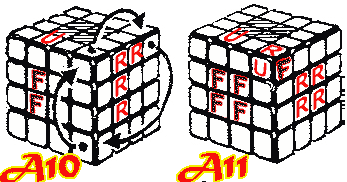8. The eighth stage: a selection of costal cubes of the bottom layer.
The basic operation looks so:
(F_D'_F' ) U'C (F D F ') UC.... А12. If to execute algorithm three times, it will return a cube in an initial condition. Having replaced In ' with on Н'с, either on В2, or on ВсНс, we shall receive set of variants of basic algorithm А12. Combining А12 with one or two rotations of external layers (examples in figure. А13-А16), completely we put in order last layer.
D [ (F D' F ') (U'C) (F D F ') (UC) ] D'.................. А13
(L D' L') (DC) (L D L') (D'C)............................... A14
D(L*2) (F D' F ') (UC)*2 (F D F ') (UC)*2 (L*2) D '....... A15
If at the final stage the situation, as in figure (
А16) cubes can on be changed will turn out Places multirunning process А16.
F (U'C) F (LLC)*2 - F' (UC) F (LLC)*2 - F R' (DC) R (UC) R' (D'C) R (U'C) F (UC)... A16